# Ranking Rows of Pandas DataFrame

To rank the rows of Pandas DataFrame we can use the `DataFrame.rank() `method which returns a rank of every respective index of a series passed. The rank is returned on the basis of position after sorting.

Example #1 :

Here we will create a DataFrame of movies and rank them based on their ratings.

 `# import the required packages  ` `import` `pandas as pd  ` ` `  `# Define the dictionary for converting to dataframe  ` `movies ``=` `{``'Name'``: [``'The Godfather'``, ``'Bird Box'``, ``'Fight Club'``], ` `         ``'Year'``: [``'1972'``, ``'2018'``, ``'1999'``], ` `         ``'Rating'``: [``'9.2'``, ``'6.8'``, ``'8.8'``]} ` ` `  `df ``=` `pd.DataFrame(movies) ` `print``(df) `

Output: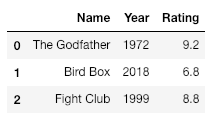`# Create a column Rating_Rank which contains ` `# the rank of each movie based on rating ` `df[``'Rating_Rank'``] ``=` `df[``'Rating'``].rank(ascending ``=` `1``) ` ` `  `# Set the index to newly created column, Rating_Rank ` `df ``=` `df.set_index(``'Rating_Rank'``) ` `print``(df) `

Output: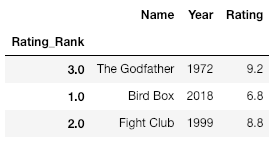`# Sort the dataFrame based on the index ` `df ``=` `df.sort_index() ` `print``(df) `

Output: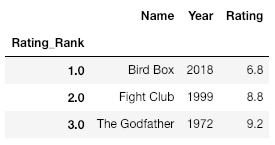Example #2
Let’s take an example of marks scored by 4 students. We will rank the students based on the highest mark they have scored.

 `# Create a dictionary with student details ` `student_details ``=` `{``'Name'``:[``'Raj'``, ``'Raj'``, ``'Raj'``, ``'Aravind'``, ``'Aravind'``, ``'Aravind'``, ` `                             ``'John'``, ``'John'``, ``'John'``, ``'Arjun'``, ``'Arjun'``, ``'Arjun'``], ` `                   ``'Subject'``:[``'Maths'``, ``'Physics'``, ``'Chemistry'``, ``'Maths'``, ``'Physics'``, ` `                             ``'Chemistry'``, ``'Maths'``, ``'Physics'``, ``'Chemistry'``, ``'Maths'``, ` `                                                           ``'Physics'``, ``'Chemistry'``], ` `                   ``'Marks'``:[``80``, ``90``, ``75``, ``60``, ``40``, ``60``, ``80``, ``55``, ``100``, ``90``, ``75``, ``70``] ` `               ``} ` ` `  `# Convert dictionary to a DataFrame ` `df ``=` `pd.DataFrame(student_details) ` `print``(df) `

Output: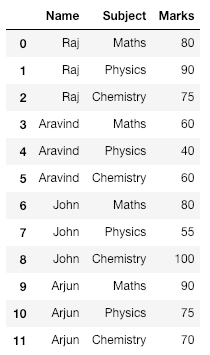`# Create a new column with Marks  ` `# ranked in descending order ` `df[``'Mark_Rank'``] ``=` `df[``'Marks'``].rank(ascending ``=` `0``) ` ` `  `# Set index to newly created column  ` `df ``=` `df.set_index(``'Mark_Rank'``) ` `print``(df) `

Output: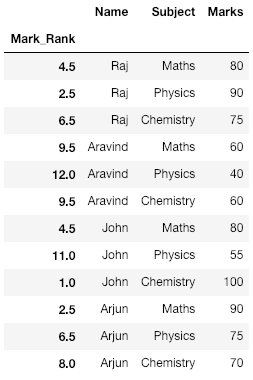`# Sort the DataFrame based on the index  ` `df ``=` `df.sort_index() ` ` `  `print``(df) `

Output: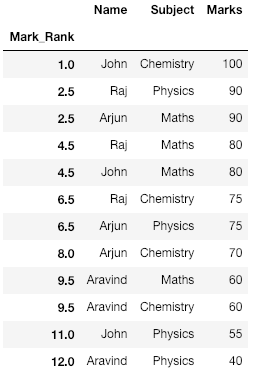Explanation:
Notice here that we have Raj and Arjun getting 90 marks each and hence they get ranked 2.5 (average of 2nd and 3rd rank i.e the two ranks they share). This can be seen for other marks in the table as well.

Whether you're preparing for your first job interview or aiming to upskill in this ever-evolving tech landscape, GeeksforGeeks Courses are your key to success. We provide top-quality content at affordable prices, all geared towards accelerating your growth in a time-bound manner. Join the millions we've already empowered, and we're here to do the same for you. Don't miss out - check it out now!

Previous
Next